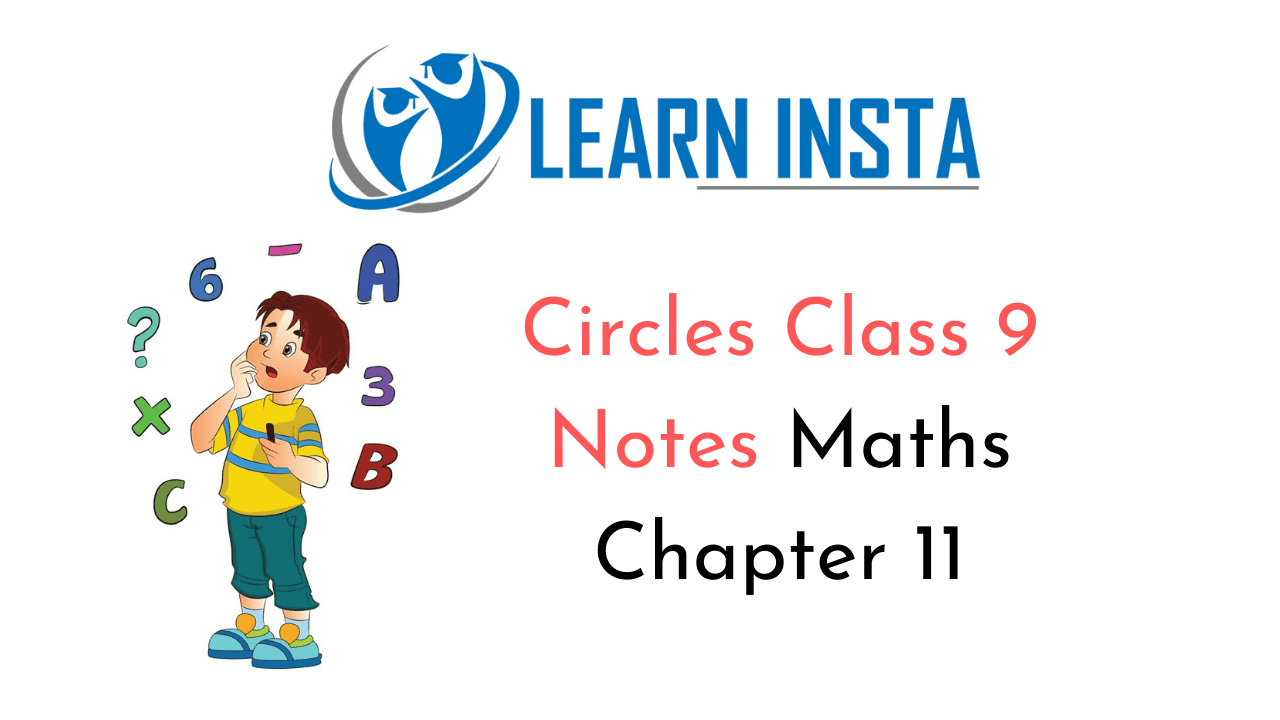On this page, you will find Circles Class 9 Notes Maths Chapter 11 Pdf free download. CBSE NCERT Class 9 Maths Notes Chapter 11 Circles will seemingly help them to revise the important concepts in less time.

## CBSE Class 9 Maths Chapter 11 Notes Circles

### Circles Class 9 Notes Understanding the Lesson

Circle is the collection of all points in a plane, which are equidistant from a fixed point in the plane. The fixed point is called the centre O and the given distance is called the radius r of the circle.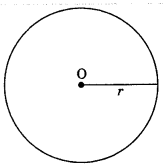Concentric circles: Circles having same centre and different radii are called concentric circles.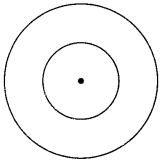Arc: A continuous piece of a circle is called an arc of the circle.

Chord: A line segment joining any two points on a circle is called the chord of the circle.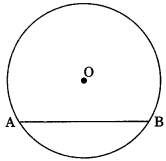Diameter: A chord passing through the centre of a circle is called the diameter of the circle.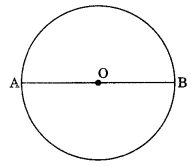• Semicircle: A diameter of a circle divides it into two equal parts which are arc. Each of these two arcs is called semicircle.
• Angle of semicircle is right angle.
• If two arcs are equal, then their corresponding, chords are also equal.

Theorem 10.1: Equal chords of a circle subtend equal angle at the centre of the circle.
Theorem 10.2: If the angles subtended by the chords of a circle at the centre are equal, then the chords are equal.
Theorem 10.3: The perpendicular drawn from centre to the chord of circle bisects the chord.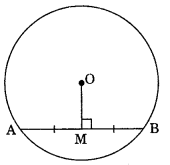Theorem 10.4: The line drawn through the centre of a circle to bisect a chord is perpendicular to the chord. Theorem 10.5: There is one and only one circle passing through three non-collinear points.
Theorem 10.6: Equal chords of circle are equidistant from centre.

Theorem 10.7: Chords equidistant from the centre of a circle are equal in length.

• If two circles intersect in two points, then the line through the centres is perpendicular to the common chord.

Theorem 10.8: The angle subtended by an arc at the centre of circle is twice the angle subtended at remaining part of circumference.
Theorem 10.9: Any two angles in the same segment of the circle are equal.
Theorem 10.10: If a line segment joining two points subtends equal angles at two other points on the same side of the line containing the line segment, the four points lie on a circle (i.e., they are concyclic).

Cyclic Quadrilateral: If all the vertices of a quadrilateral lie on the circumference of circle, then quadrilateral is called cyclic.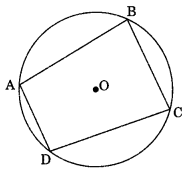Theorem 10.11: In a cyclic quadrilateral the sum of opposite angles is 180°.
Theorem 10.12: In a quadrilateral if the sum of opposite angles is 180°, then quadrilateral is cyclic.

• The exterior angle of a cyclic quadrilateral is equal to the interior opposite angle.